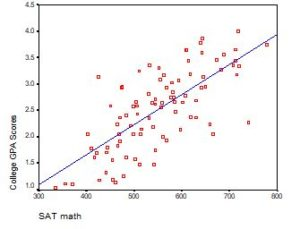# regression models

### Interpreting the Intercept in a Regression Model

February 21st, 2023 by

Interpreting the Intercept in a regression model isn’t always as straightforward as it looks.

Here’s the definition: the intercept (often labeled the constant) is the expected value of Y when all X=0. But that definition isn’t always helpful. So what does it really mean?

### Regression with One Predictor XIf X sometimes equals 0, the intercept is simply the expected value of Y at that value. In other words, it’s the mean of Y at one value of X. That’s meaningful.

If X never equals 0, then the intercept has no intrinsic meaning. You literally can’t interpret it. That’s actually fine, though. You still need that intercept to give you unbiased estimates of the slope and to calculate accurate predicted values. So while the intercept has a purpose, it’s not meaningful.

Both these scenarios are common in real data. (more…)

### When Linear Models Don’t Fit Your Data, Now What?

June 20th, 2022 by

When your dependent variable is not continuous, unbounded, and measured onan interval or ratio scale, linear models don’t fit. The data just will not meet the assumptions of linear models. But there’s good news, other models exist for many types of dependent variables.

Today I’m going to go into more detail about 6 common types of dependent variables that are either discrete, bounded, or measured on a nominal or ordinal scale and the tests that work for them instead. Some are all of these.

### Measures of Model Fit for Linear Regression Models

May 9th, 2022 by

A well-fitting regression model results in predicted values close to the observed data values.The mean model, which uses the mean for every predicted value, generally would be used if there were no useful predictor variables. The fit of a proposed
regression model should therefore be better than the fit of the mean model. (more…)

### Member Training: Difference in Differences

November 30th, 2021 byThe great majority of all regression modeling explores and tests the association between independent and dependent variables. We are not able to claim the independent variable(s) has a causal relationship with the dependent variable. There are five specific model types that allow us to test for causality. Difference in differences models are one of the five.

### Eight Ways to Detect Multicollinearity

February 25th, 2019 byMulticollinearity can affect any regression model with more than one predictor. It occurs when two or more predictor variables overlap so much in what they measure that their effects are indistinguishable.

When the model tries to estimate their unique effects, it goes wonky (yes, that’s a technical term).

So for example, you may be interested in understanding the separate effects of altitude and temperature on the growth of a certain species of mountain tree.

### Parametric or Semi-Parametric Models in Survival Analysis?

August 13th, 2018 by

It was Casey Stengel who offered the sage advice, “If you come to a fork in the road, take it.”

When you need to fit a regression model to survival data, you have to take a fork in the road. One road asks you to make a distributional assumption about your data and the other does not. (more…)